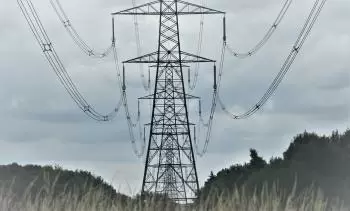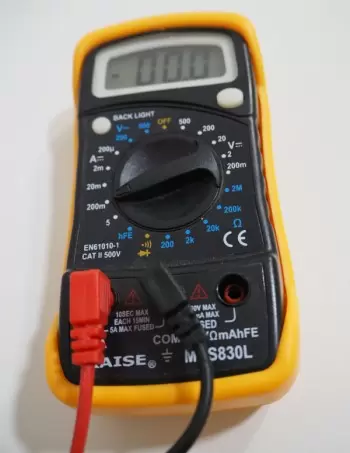# What Is Electric Current Intensity (amperage)?

The current intensity is the electrical charge that passes through a section of the conductor per second. It represents the movement of the electric charge.An electric current in a wire, where the charge carriers are electrons negatively charged, is a measure of the quantity of charge passing any point of the wire per unit of time.

A flow of positive charges (such as protons or positive ions) has the same effect in a circuit, as an equal flow of electrons in the opposite direction.

The following formula gives the amperage:

I = Q / t

Where:

• “I” represents the electric current intensity expressed in Amps (A).

• “Q” represents the electric charge expressed in Coulombs (C).

• “t” represents the time which is expressed in second units (s).

With this definition, we can say that an electric current has an intensity of 1 amp when a load of 1 colombium passes through a section of the conductor in 1 second.

## What Is the Unit to Measure the Intensity of an Electric Current?

The unit of measurement for intensity is Ampere A in the International System of Units.

The ampere unit is named after André-Marie Ampère. It is the unit of measure in measuring the amount of electrical current intensity in a circuit.

The intensity of an electric current is measured with the galvanometer. For a correct measurement, the galvanometer must be placed in series with the electrical conductor whose amperage is to be measured.An ammeter is a galvanometer calibrated in amps.

### What Are the Amp-hours Units?

An ampere-hour is a unit that measures the amount of electrical charge that flows through a storage device if it supplies a current of 1 ampere for 1 hour. An ampere-hour equals 3,600 coulombs.

## How Is the Current Intensity Generated?

Imagine two ends of the same conductor. Each lot is subjected to a different electric field with different electric potentials.

Due to this potential difference, the electrons present in the conductor experience attractive and repulsive forces. These electrical forces cause the free electrons to move.

The electrical charges move at a certain speed. We consider any section located at a point between the two extremes. A certain number of electrons per second will pass through this section, which is the same: a certain amount of electricity per second.

That amount of electric power per second is called the intensity of the electric current expressed in amps.

Two types of electric current can exist in an electrical circuit :

• Direct current (DC) is the current where electrical energy always flows in the same direction.

• Alternating current (AC) is the form of current where the charges change its sense periodically.

## What Is the Current Density?

With the current density, we can express the average quantity of electricity per unit of a conductor's bone. The current density is designated by the symbol J. In the definition of current density, we assume that current flows uniformly through the conductor cross-section.

J=I/S

J is measured in the International System units in A / m 2 (amps per square meter). However, given the conductors' dimensions, it is common to express it in A / mm2. As it is the conductor section, it is more manageable to measure in square millimeters.

## Examples of Typical Current Intensities

Here are some examples of typical current strengths in different situations:

1. In a solar panel used to generate solar energy, the current intensity can vary depending on the power and the design of the panel. Under normal conditions, the current intensity can range from 4 to 10 amps, depending on the size and efficiency of the panel.

2. In a household incandescent lamp, the typical current intensity can be from 0.5 to 2 amps (A).

3. In a mobile phone charger, the current intensity can be from 1 to 2.4 A, depending on the model.

4. In a car battery, the charging current can vary between 10 and 15 A.

5. In a residential electrical circuit, the typical current supplied by the electrical panel is 15-20A for standard outlets.

6. In an electric iron, the current intensity can be around 8 to 12 A.

7. In a medium-sized electric motor, current draw can range from a few amps to several tens of amps, depending on the load and motor design.

8. In a high-voltage transmission line, the current can reach several thousand amperes, depending on the load capacity and the transmission distance.

9. In an industrial facility, current strength can vary widely depending on the equipment and connected load, from a few amps to several hundred amps.

10. In a conventional power plant, the current intensity can be extremely high. For example, in a coal or gas power plant, the current in the generators can reach levels of several thousand amps, as large amounts of electrical power are generated to supply a large-scale distribution network.

Author:

Published: November 17, 2019
Last review: June 29, 2023